# The Mechanics of Time Value

The process of discounting future cash flows converts them into cash flows in present value terms. Conversely, the process of compounding converts present cash flows into future cash flows. There are five types of cash flows—simple cash flows, annuities, growing annuities, perpetuities, and growing perpetuities—which we discuss next.

## Simple Cash Flows

A simple cash flow is a single cash flow in a specified future time period; it can be depicted on a time line as in Figure A3.3.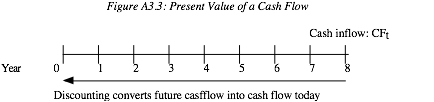where CFt = the cash flow at time t.

This cash flow can be discounted back to the present using a discount rate that reflects the uncertainty of the cash flow. Concurrently, cash flows in the present can be compounded to arrive at an expected future cash flow.

Discounting a cash flow converts it into present value dollars and enables the user to do several things. First, once cash flows are converted into present value dollars, they can be aggregated and compared. Second, if present values are estimated correctly, the user should be indifferent between the future cash flow and the present value of that cash flow. The present value of a cash flow can be written as follows

Present Value of Simple Cash Flow =where r = discount rate.

Other things remaining equal, the present value of a cash flow will decrease as the discount rate increases and continue to decrease the further into the future the cash flow occurs.

Current cash flows can be moved to the future by compounding the cash flow at the appropriate discount rate.

Future Value of Simple Cash Flow = CF0 (1 + r)t

where CF0 = cash flow now, r = discount rate. Again, the compounding effect increases with both the discount rate and the compounding period. As the length of the holding period is extended, small differences in discount rates can lead to large differences in future value.

The frequency of compounding affects both the future and present values of cash flows. In the examples just discussed, the cash flows were assumed to be discounted and compounded annually—that is, interest payments and income were computed at the end of each year, based on the balance at the beginning of the year. In some cases, however, the interest may be computed more frequently, such as on a monthly or semi-annual basis. In these cases, the present and future values may be very different from those computed on an annual basis; the stated interest rate on an annual basis can deviate significantly from the effective or true interest rate. The effective interest rate can be computed as follows:

Effective Interest Rate =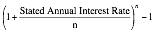where n = number of compounding periods during the year (2 = semi-annual; 12 = monthly). For instance, a 10 percent annual interest rate, if there is semi-annual compounding, works out to an effective interest rate of

Effective Interest Rate = 1.052 – 1 = 0.10125 or 10.125%

As compounding becomes continuous, the effective interest rate can be computed as follows

Effective Interest Rate = expr – 1

where exp = exponential function and r = stated annual interest rate. Table A3.2 provides the effective rates as a function of the compounding frequency.

Table A3.2 Effect of Compounding Frequency on Effective Interest Rates

 Frequency Rate t (Days) Formula Effective Annual Rate Annual 10% 1 0.10 10% Semi-annual 10% 2 (1 + 0.10/2)2 – 1 10.25% Monthly 10% 12 (1 + 0.10/12)12 – 1 10.47% Daily 10% 365 (1 + 0.10/365)365 – 1 10.5156% Continuous 10% exp0.10 – 1 10.5171%

As you can see, compounding becomes more frequent, the effective rate increases, and the present value of future cash flows decreases.

## Annuities

An annuity is a constant cash flow that occurs at regular intervals for a fixed period of time. Defining A to be the annuity, the time line for an annuity may be drawn as follows:

A                     A                     A                     A

|                       |                       |                       |

0                      1                      2                      3                      4

An annuity can occur at the end of each period, as in this time line, or at the beginning of each period. The present value of an annuity can be calculated by taking each cash flow and discounting it back to the present and then adding up the present values. Alternatively, a formula can be used in the calculation. In the case of annuities that occur at the end of each period, this formula can be written as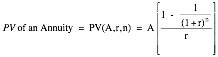where A = annuity, r = discount rate, and n = number of years. Accordingly, the notation we will use in the rest of this book for the present value of an annuity will be PV(A,r,n). In some cases, the present value of the cash flows is known and the annuity needs to be estimated. This is often the case with home and automobile loans, for example, where the borrower receives the loan today and pays it back in equal monthly installments over an extended period of time. This process of finding an annuity when the present value is known is examined here: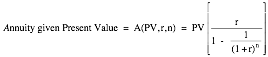Individuals or businesses who have a fixed obligation to meet or a target to meet (in terms of savings) some time in the future need to know how much they should set aside each period to reach this target. If you are given the future value and are looking for an annuity—A(FV,r,n) in terms of notation: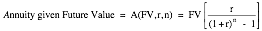The annuities considered thus far i are end-of-the-period cash flows. Both the present and future values will be affected if the cash flows occur at the beginning of each period instead of the end. To illustrate this effect, consider an annuity of \$100 at the end of each year for the next four years, with a discount rate of 10 percent.Contrast this with an annuity of \$100 at the beginning of each year for the next four years, with the same discount rate.Because the first of these annuities occurs right now and the remaining cash flows take the form of an end-of-the-period annuity over three years, the present value of this annuity can be written as follows: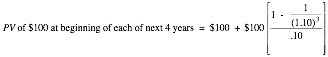In general, the present value of a beginning-of-the-period annuity over n years can be written as follows: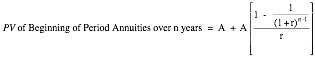This present value will be higher than the present value of an equivalent annuity at the end of each period.

## Growing Annuities

A growing annuity is a cash flow that grows at a constant rate for a specified period of time. If A is the current cash flow, and g is the expected growth rate, the time line for a growing annuity appears as follows: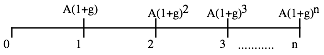Note that to qualify as a growing annuity, the growth rate in each period has to be the same as the growth rate in the prior period.

In most cases, the present value of a growing annuity can be estimated by using the following formula:The present value of a growing annuity can be estimated in all cases, but one—where the growth rate is equal to the discount rate. In that case, the present value is equal to the nominal sums of the annuities over the period, without the growth effect.

PV of a Growing Annuity for n Years (when r = g) = nA

Note also that this formulation works even when the growth rate is greater than the discount rate.

## Perpetuities and Growing Perpetuities

A perpetuity is a constant cash flow at regular intervals forever. The present value of a perpetuity can be written as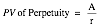where A is the perpetuity.

A growing perpetuity is a cash flow that is expected to grow at a constant rate forever. The present value of a growing perpetuity can be written as: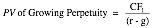where CF1 is the expected cash flow next year, g is the constant growth rate, and r is the discount rate. Although a growing perpetuity and a growing annuity share several features, the fact that a growing perpetuity lasts forever puts constraints on the growth rate. It has to be less than the discount rate for this formula to work.